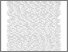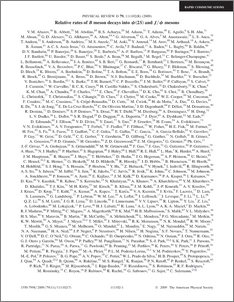# Relative rates of B meson decays into psi(2S) and J/psi mesons

Collaboration, D0 and Bertram, Iain and Borissov, Guennadi and Fox, Harald and Ratoff, Peter and Rakitin, Alexander and Love, Peter and Sopczak, Andre (2009) Relative rates of B meson decays into psi(2S) and J/psi mesons. Physical Review D, 79 (11). ISSN 1550-7998Preview
PDF
PhysRevD.79.111102.pdf - Published Version

## Abstract

We report on a study of the relative rates of B meson decays into psi(2S) and J/psi mesons using 1.3 fb^-1 of pbar p collisions at sqrt(s) = 1.96 TeV recorded by the D0 detector operating at the Fermilab Tevatron Collider. We observe the channels B^0_s -> psi(2S)phi, B^0_s -> J/psi phi, B^+/- -> psi(2S) K^+/-, and B^+/- -> J/psi K^+/- and we measure the relative branching fractions for these channels to be B(B^0_s -> psi(2S)phi)/B(B^0_s -> J/psi phi) = 0.55 +/- 0.11 (stat) +/- 0.07 (syst) +/- 0.06 (B), B(B^+/- -> psi(2S) K^+/-)/B(B^+/- -> J/psi K^+/-) = 0.65 +/- 0.04 (stat) +/- 0.03 (syst) +/- 0.07 (B) where the final error corresponds to the uncertainty in the J/psi and psi(2S) branching ratio into two muons.

Item Type:
Journal Article
Journal or Publication Title:
Physical Review D